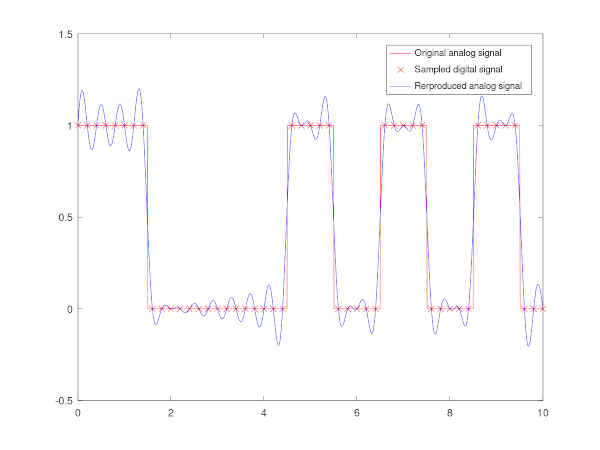## May – Digital to analog modulation

Digital signal consists of values at discrete times, whereas analog signal is continuous in time. As the orthogonal set of sinc functionssincspans the space of signals of bandwidth, an analog signal can be (uniquely) produced from a digital signal of lengthas a superposition of the sinc basis-functionssincwhereis the sampling rate of the digital signal.

The following GNU Octave function produces the analog signal from a given digital signal

%%Function returns the values at times T of the analog signal of the corresponding digital signal sampled at speed Fs.
function xb = digitaltoanalog(T, digitalsignal, Fs)
xb = [];
for t = T
xb =  [xb sum(digitalsignal.*sinc(Fs*t - (0 : length(digitalsignal) - 1)))];
end
end


The following code simulates a rectangle pulse train signal given as input, samples the input to a digital signal, and modulates the sampled digital signal back to an analog signal. As the Nyquist rate restricts the bandwidth of the signalto, information is lost in the sampling stage, because the original rectangle train has an infinite bandwidth.

pkg load signal;
close all;
clear all;

input  = @(t) pulstran(t,[0,1,5,7,9],"rectpuls") %%The original analog signal at time t.

Fs = 5; %Sampling rate.
t = 10 %Time length of the signal.
Ts = 0 : 1/Fs : t; %Sampling time instances.
x =  input(Ts); %Sampled values, i.e. the digital signal.

Ta = linspace(0, t, 1000); %Analog signal time instances for the plot.
S = digitaltoanalog(Ta, x, Fs); %Modulated analog signal.

%Plot.
plot(Ta, input(Ta), 'color', 'r');
hold on;
plot(Ts, x, 'x', 'color', 'r')
plot(Ta, S, 'color', 'b');
legend( 'Original analog signal','Sampled digital signal', 'Rerproduced analog signal')References:

• Tse, David., and Pramod. Viswanath. Fundamentals of Wireless Communication. Cambridge, U.K. ;: Cambridge University Press„ 2005. Print.